CAT  >  Test Level 1: Bar Graph

# Test Level 1: Bar Graph

Test Description

## 20 Questions MCQ Test Level-wise Tests for CAT | Test Level 1: Bar Graph

Test Level 1: Bar Graph for CAT 2023 is part of Level-wise Tests for CAT preparation. The Test Level 1: Bar Graph questions and answers have been prepared according to the CAT exam syllabus.The Test Level 1: Bar Graph MCQs are made for CAT 2023 Exam. Find important definitions, questions, notes, meanings, examples, exercises, MCQs and online tests for Test Level 1: Bar Graph below.
Solutions of Test Level 1: Bar Graph questions in English are available as part of our Level-wise Tests for CAT for CAT & Test Level 1: Bar Graph solutions in Hindi for Level-wise Tests for CAT course. Download more important topics, notes, lectures and mock test series for CAT Exam by signing up for free. Attempt Test Level 1: Bar Graph | 20 questions in 40 minutes | Mock test for CAT preparation | Free important questions MCQ to study Level-wise Tests for CAT for CAT Exam | Download free PDF with solutions
 1 Crore+ students have signed up on EduRev. Have you?
Test Level 1: Bar Graph - Question 1

### Directions: The following graph shows the performance of FANTASTIC Ltd. over the given years. Refer to the graph to answer the question that follows.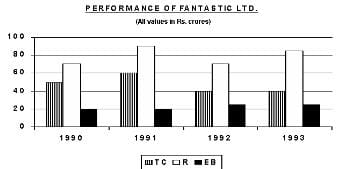Total Cost(TC) = Raw Material Cost + Labour Cost + Interest Cost Profit = Revenue (R) - Total CostT (TC) Shareholders Satisfaction = (Revenue - Total Cost)/Equity Base (EB) Q. If the raw material, labour and interest costs for 1991 were in the ratio 7 : 2 : 3, then the raw material cost for 1991 was (in Rs. crore)

Detailed Solution for Test Level 1: Bar Graph - Question 1

For 1991, Total cost = Rs. 60 crore
When we divide 60 into ratio of 7 : 2 : 3, we get Rs. 35 crore, Rs. 10 crore, Rs. 15 crore.
Therefore, the raw material cost for 1991 was Rs. 35 crore.

Test Level 1: Bar Graph - Question 2

### Directions: Study the given bar graph and answer the following question. The bar graph below shows the sales (in Rs. 000) of clothes in different regions during different quarters of a year: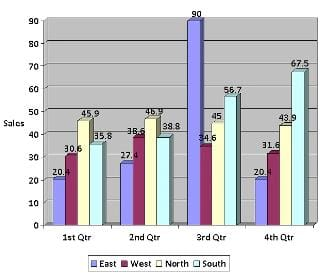Q. What were the average sales (in Rs. 000) during the second quarter?

Detailed Solution for Test Level 1: Bar Graph - Question 2

Average sales during second quarter =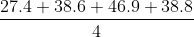= 37.925

Test Level 1: Bar Graph - Question 3

### Directions: Study the given data and answer the following question. Sales of two companies A and B over 12 months: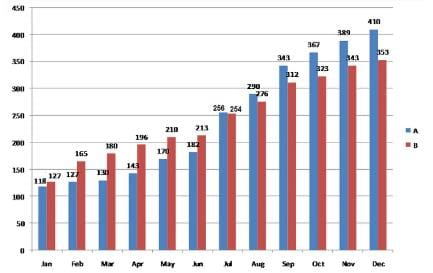Q. Which company has more sales?

Detailed Solution for Test Level 1: Bar Graph - Question 3

Total sales of company A = 118 + 127 + 130 + 143 + 170 + 182 + 256 + 290 + 343 + 367 + 389 + 410 = 2925
Total sales of company B = 127 + 165 + 180 + 196 + 210 + 213 + 254 + 276 + 312 + 323 + 343 + 353 = 2952
Sales of company B are more than sales of company A.

Test Level 1: Bar Graph - Question 4

Directions: Study the given data and answer the following question.

Sales of two companies A and B over 12 months:Q. During which quarter were the sales of company A maximum?

Detailed Solution for Test Level 1: Bar Graph - Question 4

Sales of company A during each quarter:
First quarter: Jan to Mar:
Total sales = (118 + 127 + 130) = 375
Second quarter: Apr to Jun:
Total sales = (143 + 170 + 182) = 495
Third quarter: Jul to Sept:
Total sales = (256 + 290 + 343) = 889
Fourth quarter: Oct to Dec:
Total sales = (367 + 389 + 410) = 1166
So, the sales were maximum during the last quarter.

Test Level 1: Bar Graph - Question 5

Directions: Answer the question based on the following bar graph.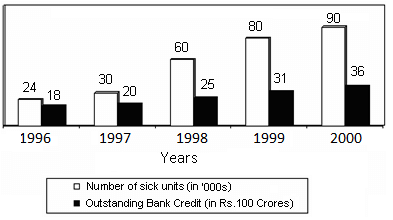Q. If the bank credit were reduced to half in the year 1997, then what would be the approximate outstanding bank credit per sick unit?

Detailed Solution for Test Level 1: Bar Graph - Question 5

New bank credit in 1997 = 1/2 of 20 × 109 = 10 × 109
Bank credit per sick unit = (10 × 109)/(30 × 1000) = Rs. 3 lakh (approx.)

Test Level 1: Bar Graph - Question 6

Directions: Answer the question based on the following bar graph.Q. What was the percentage increase in number of sick units from 1997 to 1999?

Detailed Solution for Test Level 1: Bar Graph - Question 6

Percentage increase = ((80 - 30)/30) × 100 = 166.67%

Test Level 1: Bar Graph - Question 7

Directions: Study the given bar graph and answer the following question.

Number of engineering students (in hundreds) at different institutions in different years: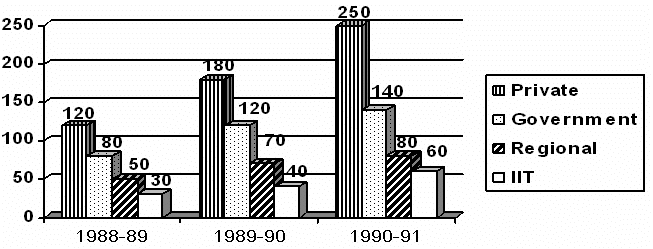Q. What was the total number of engineering students in 1989-90?

Detailed Solution for Test Level 1: Bar Graph - Question 7

Total number of engineering students in 1989-90 = (180 + 120 + 70 + 40) × 100 = 410 × 100 = 41,000

Test Level 1: Bar Graph - Question 8

Directions: Study the given bar graph and answer the following question.

Number of engineering students (in hundreds) at different institutions in different years:Q. The total number of engineering students in 1991-92, assuming a 10% reduction in the number over the previous year, was

Detailed Solution for Test Level 1: Bar Graph - Question 8

Total number of engineering students in 1990-91 = (250 + 140 + 80 + 60)  100
= 530  100 = 53,000
Therefore, total number of engineering students in 1991-92 = 53,000  0.90 = 47,700
None of the options gives the required figure.

Test Level 1: Bar Graph - Question 9

Directions: Study the given information and answer the following question.
The bar chart given below shows the production of different dress materials (in hundreds) from 6 cities during the years 2015 and 2016.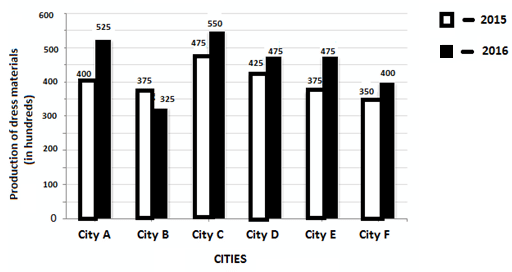Q. The average production of dress materials in cities A, B and C in 2016 is what percentage of average production of dress materials in cities D, E and F in 2015 (in approximation)?

Detailed Solution for Test Level 1: Bar Graph - Question 9

In 2016, average production of dress materials in cities A, B and C =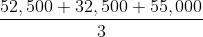= 1,40,000/3
In 2015, average production of dress materials in cities D, E and F =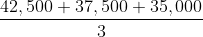= 1,15,000/3
Required percentage =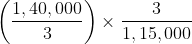× 100 = 121.73%

Test Level 1: Bar Graph - Question 10

Directions: Study the given bar graph and answer the following question.
The bar graph below shows the sales (in Rs. 000) of clothes in different regions during different quarters of a year:Q. What is the approximate average sale of all regions in the first quarter (in 000's)?

Detailed Solution for Test Level 1: Bar Graph - Question 10

Sales of East region in the first quarter = 20.4 (in 000's)
Sales of West region in the first quarter = 30.6 (in 000's)
Sales of North region in the first quarter = 45.9 (in 000's)
Sales of South region in the first quarter = 35.8 (in 000's)

Average sales of all regions in the first quarter =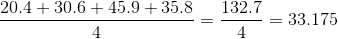(in 000's)

Test Level 1: Bar Graph - Question 11

Directions: Study the given bar graph and answer the following question.
The bar graph below shows the sales (in Rs. 000) of clothes in different regions during different quarters of a year:Q. What was the range of sales (in Rs. 000) over the four quarters in the east region?

Detailed Solution for Test Level 1: Bar Graph - Question 11

Range of sales = Maximum sales in east region - Minimum sales in east region = 90 - 20.4 = 69.6

Test Level 1: Bar Graph - Question 12

Directions: Study the given data and answer the following question.

Sales of two companies A and B over 12 months:Q. What was the percentage increase in sales of company A from March to April?

Detailed Solution for Test Level 1: Bar Graph - Question 12

Sales in March = 130
Sales in April = 143
So, percentage increase =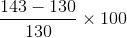= 10%

Test Level 1: Bar Graph - Question 13

Directions: Answer the question based on the following bar graph.Q. What was the difference between outstanding bank credits in 1997 and 1998?

Detailed Solution for Test Level 1: Bar Graph - Question 13

Difference = (25 - 20) × 100 crore = Rs. 500 crore

Test Level 1: Bar Graph - Question 14

Directions: Answer the question based on the following bar graph.Q. In 1999, what was the approximate average outstanding bank credit per sick unit?

Detailed Solution for Test Level 1: Bar Graph - Question 14

verage bank credit per sick unit = (31 × 109)/(80 × 1000) = Rs. 4 lakh (approx.)

Test Level 1: Bar Graph - Question 15

Directions: Study the given bar graph and answer the following question.

Number of engineering students (in hundreds) at different institutions in different years:Q. The growth rate of the number of students in government engineering colleges compared to that in private engineering colleges between 1988-89 and 1989-90 was

Detailed Solution for Test Level 1: Bar Graph - Question 15

Growth rate of the number of students in government engineering colleges between 1988-89 and 1989-90 =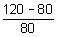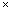100

= 50%
Growth rate of the number of students in private engineering colleges between 1988-89 and 1989-90 =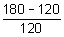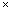100 = 50%
Hence, the growth rate was equal.

Test Level 1: Bar Graph - Question 16

Directions: Study the given information and answer the following question.

The bar chart given below shows the production of different dress materials (in hundreds) from 6 cities during the years 2015 and 2016.Q. What is the difference between the total production of dress materials in cities A, C and E together, and the total production of dress materials in cities B, D and F for the year 2016?

Detailed Solution for Test Level 1: Bar Graph - Question 16

Total production of dress materials in cities A, C and E together = 52,500 + 55,000 + 47,500 = 1,55,000
Total production of dress materials in cities B, D and F together = 32,500 + 47,500 + 40,000 = 1,20,000
Required difference = 1,55,000 – 1,20,000 = 35,000

Test Level 1: Bar Graph - Question 17

Directions: Study the given information and answer the following question.

The bar chart given below shows the production of different dress materials (in hundreds) from 6 cities during the years 2015 and 2016.Q. The total production of dress materials in City E and City F for the year 2015 is what percent of the total production of dress materials in City C and City D for the same year?

Detailed Solution for Test Level 1: Bar Graph - Question 17

In 2015, Production of dress materials in City E and City F = 37,500 + 35,000 = 72,500
In 2015, Production of dress materials in City C and City D = 47,500 + 42,500 = 90,000
Required percentage =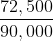100 = 80.55%

Test Level 1: Bar Graph - Question 18

Directions: Answer the question based on the information given below.
The following chart shows the runs scored by Dravid in the year 2006-07.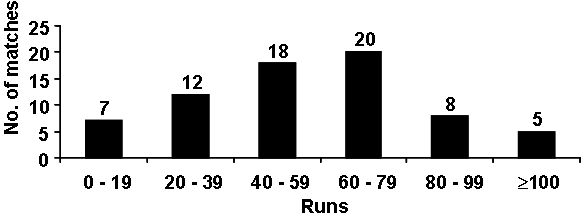Best = Highest score in any match

Average = Total runs scored/Number of outs

Q. If Dravid was out in all the innings he played, what is the minimum possible average can he have? (If his best is 118)

Detailed Solution for Test Level 1: Bar Graph - Question 18

Minimum possible number of runs
= 70 + 1220 + 1840 + 2060 + 880 + 4100 + 118 = 3318.
So, average =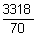= 47.4.

Test Level 1: Bar Graph - Question 19

Directions: Answer the question based on the information given below.

The following chart shows the runs scored by Dravid in the year 2006-07.Best = Highest score in any match

Average = Total runs scored/Number of outs

Detailed Solution for Test Level 1: Bar Graph - Question 19

Average =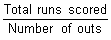, it will become maximum if total runs scored is maximum.
The maximum possible runs he can score is19 + 1239 + 1859 + 2079 + 899 + 5120 = 4635 (Since best is 120).
Total number of outs = 7 + 12 + 18 + 20 + 8 + 5 = 70.
So, Average =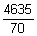= 66.21 ≈ 66.

Test Level 1: Bar Graph - Question 20

Directions: Study the given graph carefully and answer the following question.

The graph below shows the numbers of students appearing for and qualifying in a scholarship exam from various schools: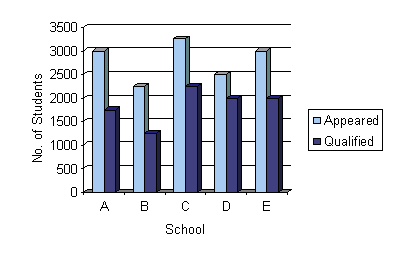Detailed Solution for Test Level 1: Bar Graph - Question 20

Total number of students who appeared for the exam from schools A, B and C = 3000 + 3250 + 2250 = 8500
Total number of students who appeared for the exam from schools D and E = 5500
Difference = 8500 - 5500 = 3000
Required percentage = (3000 × 100)/5500 = 54.5%

## Level-wise Tests for CAT

5 docs|272 tests
 Use Code STAYHOME200 and get INR 200 additional OFF Use Coupon Code
Information about Test Level 1: Bar Graph Page
In this test you can find the Exam questions for Test Level 1: Bar Graph solved & explained in the simplest way possible. Besides giving Questions and answers for Test Level 1: Bar Graph, EduRev gives you an ample number of Online tests for practice

## Level-wise Tests for CAT

5 docs|272 tests

### How to Prepare for CAT

Read our guide to prepare for CAT which is created by Toppers & the best Teachers